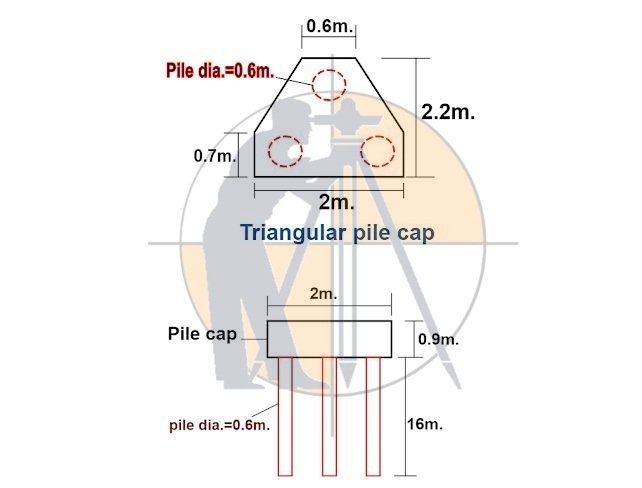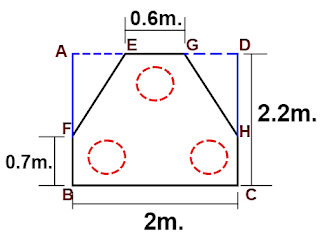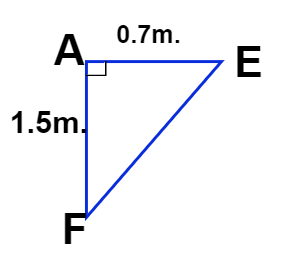Breaking News
Home / Civil Engineering / Concrete Work / How To Calculate The Volume Of Concrete Triangular Pile Cap Having 3-Piles

# How To Calculate The Volume Of Concrete Triangular Pile Cap Having 3-Piles

## How To Calculate The Volume Of Concrete Triangular Pile Cap Having 3-Piles

Let us calculate the volume of concrete in a triangular pile foundation having 3 nos. of the pile, as shown below.### Given data:

Pile diameter = 0.6m.( d )

No. of piles = 3 nos.

Length of pile = 16m. (h )

Depth of the pile cap = 0.9m.(D )

### The volume of concrete in the piles

= 3nos.× πr2 h

Here,

r = radius of the pile.

= d ÷ 2

= 0.6m ÷ 2

### = 0.3m.

h = length of the pile.

### The volume of concrete in piles

= 3nos. × 3.142 × (0.3m)2 × 16m.

### The volume of concrete in the pile cap :

The volume of  pile cap concrete

=  Surface area (A ) × depth ( D )

First, let us calculate the sectional area (A1) of rectangle ABCD, as shown in the below drawing.Area of rectangle ABCD (A1)

=L × B

= 2.2m × 2.0m

### = 4.4 sqm.

To get the surface area ( A) of the pile cap, we have to deduct the area of triangles AEF & GDH from the rectangle area.

### Here,

Triangle AEF = triangle GDH

### Area of triangle AEF

= 0.5 × base × height.

= 0.5 × side AE × side AF

Side AE

= [ ( 1/2 ×side BC ) – (1/2 × side EG )]

=  [ ( 1/2 ×2m. ) – (1/2 × 0.6m. )]

= [ 1m – 0.3 m ]

### = 0.7m.

Side AF

= side AB – side FB

= 2.2m – 0.7m

### Basic Rule For Design Of Concrete Column

I have redrawn the triangle, with the calculated length of the sides AF & AE as shown below.Area of triangle AEF

= 0.5 × side AE × side AF

= 0.5 × 0.7m × 1.5m.

### = 0.525 sqm.

The surface area of the pile cap (A )

= [area of rectangle ABCD – (2nos. × area of a triangle AEF )]

= [4.4 sqm. – (2nos. × 0.525sqm.)]

= [4.4 sqm. – 1.05 sqm.]

### = 3.35 sqm.

Now, the concrete vol. of pile cap

= surface area (A ) × depth (D )

= 3.35 sqm. × 0.9m.

### The total concrete volume of 3-pile foundation

= The vol. of concrete in pile cap + total vol. of concrete in piles.

= 3.015 cum + 13.57 cum.

## . Land Surveying & Architects

### THANKS.